# OpenCV学习笔记-分水岭算法

## 一、分水岭算法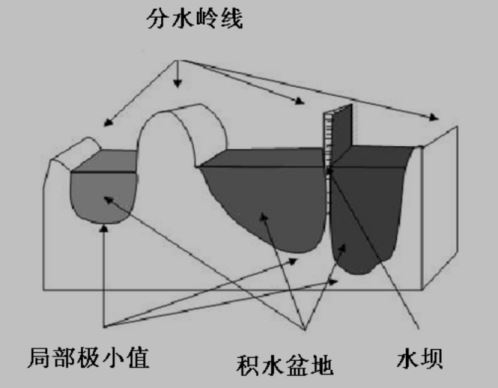## 二、代码

1、我们找到硬币的近似估计

gray = cv.cvtColor(blurred, cv.COLOR_BGR2GRAY)
ret, thresh = cv.threshold(gray, 0, 255, cv.THRESH_BINARY | cv.THRESH_OTSU)
cv.imshow( 'thresh', thresh)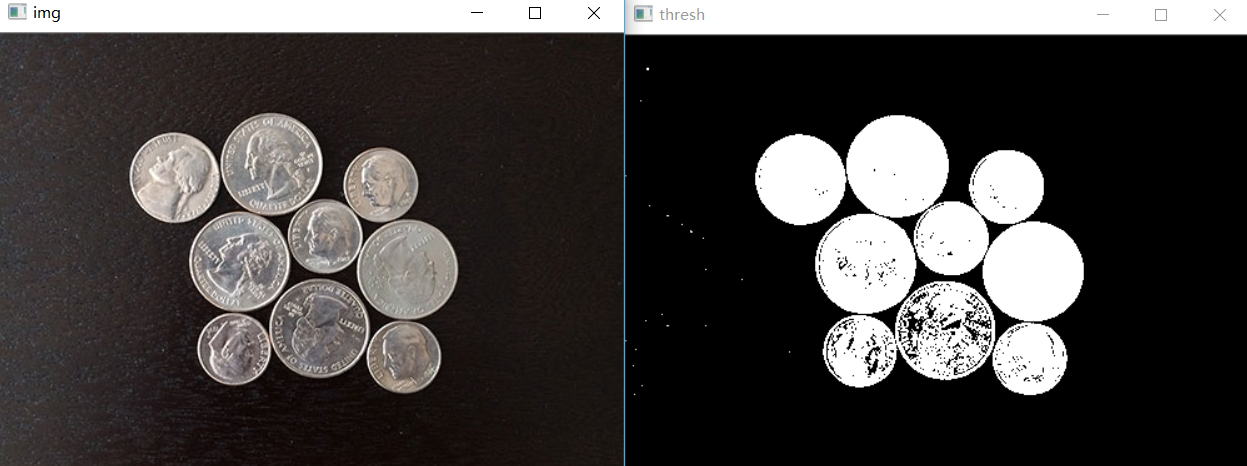#去噪声
blurred = cv.pyrMeanShiftFiltering(img, 10, 100)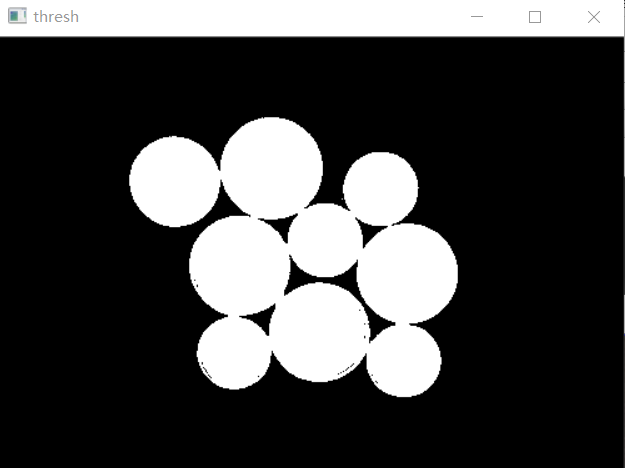kernel = cv.getStructuringElement(cv.MORPH_RECT, (3, 3))
opening = cv.morphologyEx(thresh, cv.MORPH_OPEN, kernel, iterations=2)
cv.imshow('opening ', opening)
sure_bg = cv.dilate(opening, kernel, iterations=3)
cv.imshow('mor-opt', sure_bg)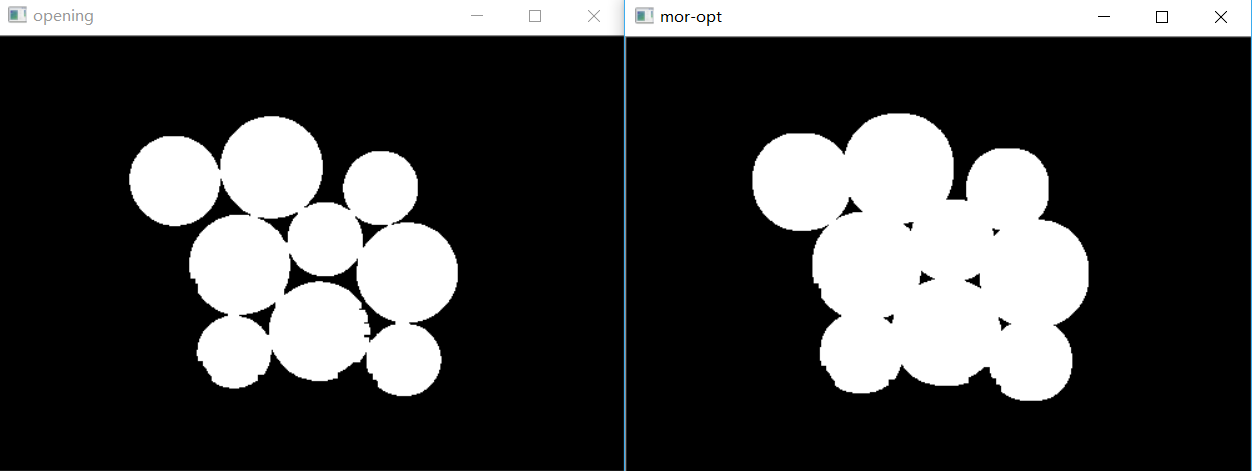#距离变换
dist = cv.distanceTransform(opening, cv.DIST_L2, 3)
dist_output = cv.normalize(dist, 0, 1.0, cv.NORM_MINMAX)
cv.imshow('distance-t', dist_output * 50)

ret, surface = cv.threshold(dist, dist.max()*0.6, 255, cv.THRESH_BINARY)
cv.imshow('surface', surface)

#Finding unknown region
surface_fg = np.uint8(surface)
cv.imshow('surface_bin', surface_fg)
unknown = cv.subtract(sure_bg,surface_fg)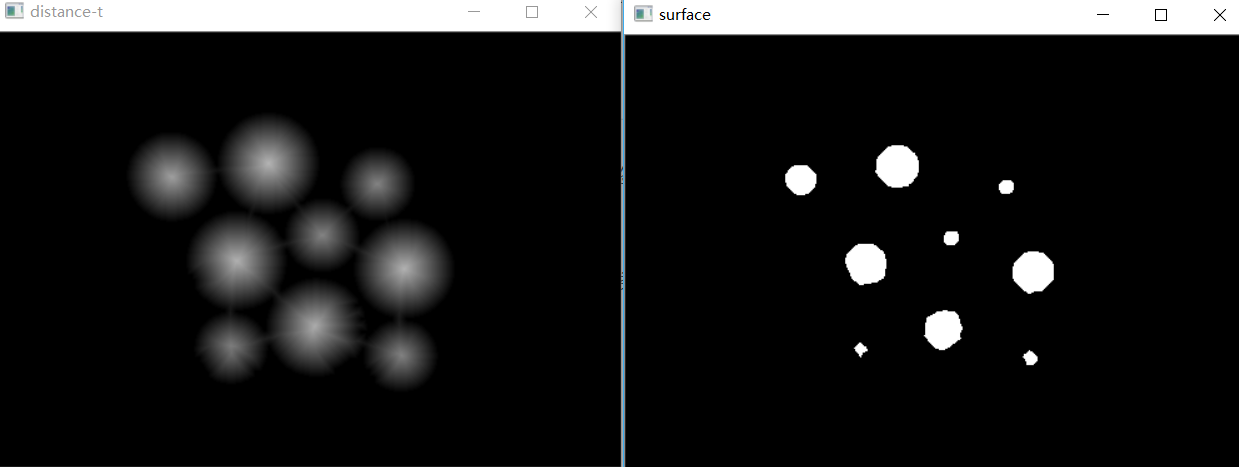# Marker labelling
ret, markers = cv.connectedComponents(surface_fg)

# Add one to all labels so that sure background is not 0, but 1
markers = markers + 1

# now,make thre region of unknown with zero
markers[unknown==255] = 0


markers = cv.watershed(img, markers)
img[markers==-1] = [255, 0, 0]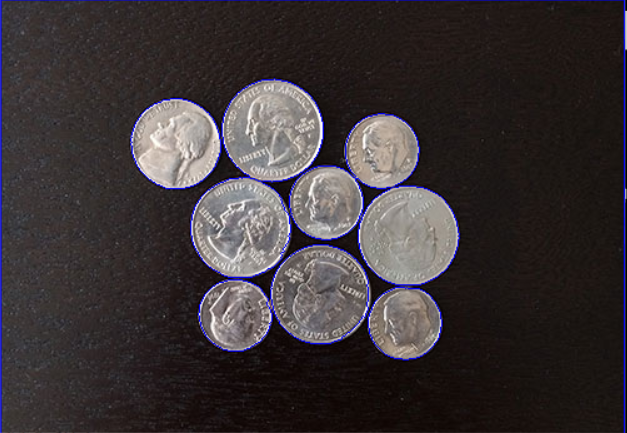import cv2 as cv
import numpy as np
from matplotlib import pyplot as plt

def watershed_demo(img):
print(img.shape)
#去噪声
blurred = cv.pyrMeanShiftFiltering(img, 10, 100)
# gray\binary image
gray = cv.cvtColor(blurred, cv.COLOR_BGR2GRAY)
ret, thresh = cv.threshold(gray, 0, 255, cv.THRESH_BINARY | cv.THRESH_OTSU)
cv.imshow('thresh', thresh)

# 有很多的黑点，所以我们去黑点噪声
kernel = cv.getStructuringElement(cv.MORPH_RECT, (3, 3))
opening = cv.morphologyEx(thresh, cv.MORPH_OPEN, kernel, iterations=2)
cv.imshow('opening ', opening)
sure_bg = cv.dilate(opening, kernel, iterations=3)
cv.imshow('mor-opt', sure_bg)

#距离变换
dist = cv.distanceTransform(opening, cv.DIST_L2, 3)
dist_output = cv.normalize(dist, 0, 1.0, cv.NORM_MINMAX)
cv.imshow('distance-t', dist_output * 50)

ret, surface = cv.threshold(dist, dist.max()*0.6, 255, cv.THRESH_BINARY)
cv.imshow('surface', surface)

#Finding unknown region
surface_fg = np.uint8(surface)
cv.imshow('surface_bin', surface_fg)
unknown = cv.subtract(sure_bg,surface_fg)

# Marker labelling
ret, markers = cv.connectedComponents(surface_fg)

# Add one to all labels so that sure background is not 0, but 1
markers = markers + 1

# now,make thre region of unknown with zero
markers[unknown==255] = 0

markers = cv.watershed(img, markers)
img[markers==-1] = [255, 0, 0]

cv.imshow('result', img)

cv.namedWindow('img',cv.WINDOW_AUTOSIZE)
cv.imshow('img',img)

watershed_demo(img)

cv.waitKey(0)
cv.destroyAllWindows()

05-0803-03
11-19
10-087925
09-112490
08-271万+
01-1325
09-05225
03-06
10-185万+
11-151304
12-21548
08-286785
01-29461
06-255536
03-141万+
05-244911
08-025142